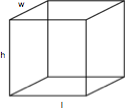## ASVAB Math Knowledge Practice Test 317240

Question 2 of 5
###### Cubes

A cube is a rectangular solid box with a height (h), length (l), and width (w). The volume is h x l x w and the surface area is 2lw x 2wh + 2lh.The dimensions of this cube are height (h) = 6, length (l) = 8, and width (w) = 9. What is the surface area?

 106 40 28 348